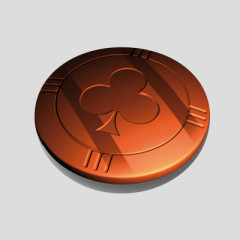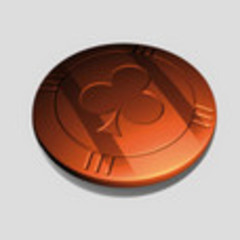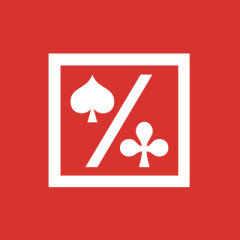В целях облегчения навигации по сайту используются Cookie-файлы. Продолжая просмотр сайта, вы принимаете указанные Cookie-файлы. Для получения дополнительной информации и изменения настроек ознакомьтесь с политикой Cookie и политикой конфиденциальности.

# [HM1] Straight flash KA234

•Бронза
На форуме с: 20.12.2008
HM2 / Reports / Hand at shutdown
Одномастная комбинация KA234 показывается как straight flash. Дмаю это неправильно, но моего английского не хватит, чтобы с ними переписываться. Может кто-нибудь сделает это за меня. Мелочь, но не правильно ...
• 15 ответов
•Модератор
Модератор
На форуме с: 18.06.2008
нужен как минимум оригинал руки и скриншот.
•Бронза
На форуме с: 20.12.2008
Titan Poker Owensville 0.25/0.50, hand converted by the iPoker Converter at Talking-Poker
Visit Hand HQ to purchase hand histories from a range of sites, game types and levels.

saw flop | saw showdown

Button Button (\$19.49)
SB (\$58.34)
CO (\$8.13)
UTG UTG (\$5)
UTG+1 (\$15.69)
MP (\$6.75)
MP MP (\$44.45)
CO-1 Hero (\$15.37)
CO (\$19.85)

Preflop: Hero is in the CO-1 with A 4
1 fold, MP calls 0.25, Hero calls 0.25, 1 fold, Button calls 0.25, 1 fold, UTG checks.

Flop (1.10) 2 J 3
UTG bets 0.25, MP calls 0.25, Hero raises to 0.50, 1 fold, UTG calls 0.25, MP calls 0.25.

Turn (2.60) T
UTG bets 0.50, 1 fold, Hero calls 0.50.

River (3.60) K
UTG bets 0.50, Hero raises to 1, UTG calls 0.50

Hero shows A 4
UTG shows 3 7

Hero wins 5.60 with Flush (Diamonds) with Ace high
•Серебро
На форуме с: 03.09.2009
Оригинал пользователя stanisluv
нужен как минимум оригинал руки и скриншот.
Оригинал пользователя schizm
Titan Poker Owensville 0.25/0.50, hand converted by the iPoker Converter at Talking-Poker
Visit Hand HQ to purchase hand histories from a range of sites, game types and levels.

просили же оригинал, а не отконвертированную руку.
•Бронза
На форуме с: 20.12.2008
<?xml version="1.0" encoding="utf-8"?><session sessioncode="2929953081">
<general>
<mode>real</mode>
<gametype>Holdem L \$0.25/\$0.50</gametype>
<tablename>Owensville</tablename>
<duration>00:46</duration>
<gamecount>39</gamecount>
<startdate>2010-12-23 15:27:00</startdate>
<currency>USD</currency>
<nickname>shizmus</nickname>
<bets>\$9.45</bets>
<wins>\$12.54</wins>
<chipsin>15</chipsin>
<chipsout>18.09</chipsout>
<ipoints>11.10</ipoints>

</general>
<game gamecode="2564622895">
<general>
<startdate>2010-12-23 16:01:23</startdate>
<players>
<player seat="1" name="milleyone" chips="\$44.45" dealer="0" win="\$0" bet="\$0.75" /><player seat="2" name="shizmus" chips="\$15.37" dealer="0" win="\$5.32" bet="\$2.25" /><player seat="3" name="MaTool" chips="\$19.85" dealer="0" win="\$0" bet="\$0" /><player seat="4" name="shar29" chips="\$19.49" dealer="1" win="\$0" bet="\$0.25" /><player seat="5" name="AlexBcn30" chips="\$58.34" dealer="0" win="\$0" bet="\$0.10" /><player seat="6" name="Amnion" chips="\$8.13" dealer="0" win="\$0" bet="\$0" /><player seat="7" name="mikegreeny" chips="\$5.90" dealer="0" win="\$0" bet="\$0" /><player seat="8" name="Veloraptori" chips="\$5" dealer="0" win="\$0" bet="\$2.25" /><player seat="9" name="Basmach" chips="\$15.69" dealer="0" win="\$0" bet="\$0" /><player seat="10" name="sertano50" chips="\$6.75" dealer="0" win="\$0" bet="\$0" />
</players>
</general>
<round no="0">
<action no="1" player="AlexBcn30" type="1" sum="\$0.10" cards="[cards]"/><action no="3" player="Veloraptori" type="2" sum="\$0.25" cards="[cards]"/>
</round>
<round no="1">
<cards type="Pocket" player="milleyone">X X</cards><action no="5" player="milleyone" type="3" sum="\$0.25" cards=""/><cards type="Pocket" player="shizmus">DA D4</cards><action no="6" player="shizmus" type="3" sum="\$0.25" cards=""/><cards type="Pocket" player="MaTool">X X</cards><action no="7" player="MaTool" type="0" sum="\$0" cards=""/><cards type="Pocket" player="shar29">X X</cards><action no="8" player="shar29" type="3" sum="\$0.25" cards=""/><cards type="Pocket" player="AlexBcn30">X X</cards><action no="9" player="AlexBcn30" type="0" sum="\$0" cards=""/><action no="2" player="mikegreeny" type="9" sum="\$0" cards=""/><cards type="Pocket" player="Veloraptori">C3 H7</cards><action no="10" player="Veloraptori" type="4" sum="\$0" cards=""/><cards type="Pocket" player="Basmach">X X</cards><action no="4" player="Basmach" type="0" sum="\$0" cards=""/>
</round>
<round no="2">
<cards type="Flop" player="">D2 HJ D3</cards><action no="12" player="milleyone" type="3" sum="\$0.25" cards=""/><action no="16" player="milleyone" type="3" sum="\$0.25" cards=""/><action no="13" player="shizmus" type="23" sum="\$0.50" cards=""/><action no="14" player="shar29" type="0" sum="\$0" cards=""/><action no="11" player="Veloraptori" type="5" sum="\$0.25" cards=""/><action no="15" player="Veloraptori" type="3" sum="\$0.25" cards=""/>
</round>
<round no="3">
<cards type="Turn" player="">C10</cards><action no="18" player="milleyone" type="0" sum="\$0" cards=""/><action no="19" player="shizmus" type="3" sum="\$0.50" cards=""/><action no="17" player="Veloraptori" type="5" sum="\$0.50" cards=""/>
</round>
<round no="4">
<cards type="River" player="">DK</cards><action no="21" player="shizmus" type="23" sum="\$1" cards=""/><action no="20" player="Veloraptori" type="5" sum="\$0.50" cards=""/><action no="22" player="Veloraptori" type="3" sum="\$0.50" cards=""/>
</round>

</game><?xml version="1.0" encoding="utf-8"?>
•Бронза
На форуме с: 20.12.2008
<game gamecode="2564622895">
<general>
<startdate>2010-12-23 16:01:23</startdate>
<players>
<player seat="1" name="milleyone" chips="\$44.45" dealer="0" win="\$0" bet="\$0.75" /><player seat="2" name="shizmus" chips="\$15.37" dealer="0" win="\$5.32" bet="\$2.25" /><player seat="3" name="MaTool" chips="\$19.85" dealer="0" win="\$0" bet="\$0" /><player seat="4" name="shar29" chips="\$19.49" dealer="1" win="\$0" bet="\$0.25" /><player seat="5" name="AlexBcn30" chips="\$58.34" dealer="0" win="\$0" bet="\$0.10" /><player seat="6" name="Amnion" chips="\$8.13" dealer="0" win="\$0" bet="\$0" /><player seat="7" name="mikegreeny" chips="\$5.90" dealer="0" win="\$0" bet="\$0" /><player seat="8" name="Veloraptori" chips="\$5" dealer="0" win="\$0" bet="\$2.25" /><player seat="9" name="Basmach" chips="\$15.69" dealer="0" win="\$0" bet="\$0" /><player seat="10" name="sertano50" chips="\$6.75" dealer="0" win="\$0" bet="\$0" />
</players>
</general>
<round no="0">
<action no="1" player="AlexBcn30" type="1" sum="\$0.10" cards="[cards]"/><action no="3" player="Veloraptori" type="2" sum="\$0.25" cards="[cards]"/>
</round>
<round no="1">
<cards type="Pocket" player="milleyone">X X</cards><action no="5" player="milleyone" type="3" sum="\$0.25" cards=""/><cards type="Pocket" player="shizmus">DA D4</cards><action no="6" player="shizmus" type="3" sum="\$0.25" cards=""/><cards type="Pocket" player="MaTool">X X</cards><action no="7" player="MaTool" type="0" sum="\$0" cards=""/><cards type="Pocket" player="shar29">X X</cards><action no="8" player="shar29" type="3" sum="\$0.25" cards=""/><cards type="Pocket" player="AlexBcn30">X X</cards><action no="9" player="AlexBcn30" type="0" sum="\$0" cards=""/><action no="2" player="mikegreeny" type="9" sum="\$0" cards=""/><cards type="Pocket" player="Veloraptori">C3 H7</cards><action no="10" player="Veloraptori" type="4" sum="\$0" cards=""/><cards type="Pocket" player="Basmach">X X</cards><action no="4" player="Basmach" type="0" sum="\$0" cards=""/>
</round>
<round no="2">
<cards type="Flop" player="">D2 HJ D3</cards><action no="12" player="milleyone" type="3" sum="\$0.25" cards=""/><action no="16" player="milleyone" type="3" sum="\$0.25" cards=""/><action no="13" player="shizmus" type="23" sum="\$0.50" cards=""/><action no="14" player="shar29" type="0" sum="\$0" cards=""/><action no="11" player="Veloraptori" type="5" sum="\$0.25" cards=""/><action no="15" player="Veloraptori" type="3" sum="\$0.25" cards=""/>
</round>
<round no="3">
<cards type="Turn" player="">C10</cards><action no="18" player="milleyone" type="0" sum="\$0" cards=""/><action no="19" player="shizmus" type="3" sum="\$0.50" cards=""/><action no="17" player="Veloraptori" type="5" sum="\$0.50" cards=""/>
</round>
<round no="4">
<cards type="River" player="">DK</cards><action no="21" player="shizmus" type="23" sum="\$1" cards=""/><action no="20" player="Veloraptori" type="5" sum="\$0.50" cards=""/><action no="22" player="Veloraptori" type="3" sum="\$0.50" cards=""/>
</round>

</game>
•Модератор
Модератор
На форуме с: 18.06.2008
А скриншот репорта? Там же этих репортов дофига и больше.
•Бронза
На форуме с: 20.12.2008•Модератор
Модератор
На форуме с: 18.06.2008
не получается импортировать руку которую ты запостировал.Видать нужен весь файл с руками сыгранными за тем столом(т.е. 2929953081.xml).
•Бронза
На форуме с: 20.12.2008
Вот с заголовком сессии. Должно импортироваться, а я эту руку импортировала их HM1. Весь файл выложить не могу, надо опять на каком-то файлообменнике регистрироваться.

<?xml version="1.0" encoding="utf-8"?><session sessioncode="2929953081">
<general>
<mode>real</mode>
<gametype>Holdem L \$0.25/\$0.50</gametype>
<tablename>Owensville</tablename>
<duration>00:46</duration>
<gamecount>39</gamecount>
<startdate>2010-12-23 15:27:00</startdate>
<currency>USD</currency>
<nickname>shizmus</nickname>
<bets>\$9.45</bets>
<wins>\$12.54</wins>
<chipsin>15</chipsin>
<chipsout>18.09</chipsout>
<ipoints>11.10</ipoints>

</general>
<game gamecode="2564622895">
<general>
<startdate>2010-12-23 16:01:23</startdate>
<players>
<player seat="1" name="milleyone" chips="\$44.45" dealer="0" win="\$0" bet="\$0.75" /><player seat="2" name="shizmus" chips="\$15.37" dealer="0" win="\$5.32" bet="\$2.25" /><player seat="3" name="MaTool" chips="\$19.85" dealer="0" win="\$0" bet="\$0" /><player seat="4" name="shar29" chips="\$19.49" dealer="1" win="\$0" bet="\$0.25" /><player seat="5" name="AlexBcn30" chips="\$58.34" dealer="0" win="\$0" bet="\$0.10" /><player seat="6" name="Amnion" chips="\$8.13" dealer="0" win="\$0" bet="\$0" /><player seat="7" name="mikegreeny" chips="\$5.90" dealer="0" win="\$0" bet="\$0" /><player seat="8" name="Veloraptori" chips="\$5" dealer="0" win="\$0" bet="\$2.25" /><player seat="9" name="Basmach" chips="\$15.69" dealer="0" win="\$0" bet="\$0" /><player seat="10" name="sertano50" chips="\$6.75" dealer="0" win="\$0" bet="\$0" />
</players>
</general>
<round no="0">
<action no="1" player="AlexBcn30" type="1" sum="\$0.10" cards="[cards]"/><action no="3" player="Veloraptori" type="2" sum="\$0.25" cards="[cards]"/>
</round>
<round no="1">
<cards type="Pocket" player="milleyone">X X</cards><action no="5" player="milleyone" type="3" sum="\$0.25" cards=""/><cards type="Pocket" player="shizmus">DA D4</cards><action no="6" player="shizmus" type="3" sum="\$0.25" cards=""/><cards type="Pocket" player="MaTool">X X</cards><action no="7" player="MaTool" type="0" sum="\$0" cards=""/><cards type="Pocket" player="shar29">X X</cards><action no="8" player="shar29" type="3" sum="\$0.25" cards=""/><cards type="Pocket" player="AlexBcn30">X X</cards><action no="9" player="AlexBcn30" type="0" sum="\$0" cards=""/><action no="2" player="mikegreeny" type="9" sum="\$0" cards=""/><cards type="Pocket" player="Veloraptori">C3 H7</cards><action no="10" player="Veloraptori" type="4" sum="\$0" cards=""/><cards type="Pocket" player="Basmach">X X</cards><action no="4" player="Basmach" type="0" sum="\$0" cards=""/>
</round>
<round no="2">
<cards type="Flop" player="">D2 HJ D3</cards><action no="12" player="milleyone" type="3" sum="\$0.25" cards=""/><action no="16" player="milleyone" type="3" sum="\$0.25" cards=""/><action no="13" player="shizmus" type="23" sum="\$0.50" cards=""/><action no="14" player="shar29" type="0" sum="\$0" cards=""/><action no="11" player="Veloraptori" type="5" sum="\$0.25" cards=""/><action no="15" player="Veloraptori" type="3" sum="\$0.25" cards=""/>
</round>
<round no="3">
<cards type="Turn" player="">C10</cards><action no="18" player="milleyone" type="0" sum="\$0" cards=""/><action no="19" player="shizmus" type="3" sum="\$0.50" cards=""/><action no="17" player="Veloraptori" type="5" sum="\$0.50" cards=""/>
</round>
<round no="4">
<cards type="River" player="">DK</cards><action no="21" player="shizmus" type="23" sum="\$1" cards=""/><action no="20" player="Veloraptori" type="5" sum="\$0.50" cards=""/><action no="22" player="Veloraptori" type="3" sum="\$0.50" cards=""/>
</round>

</game>
</session>
•Супер-модератор
Супер-модератор
На форуме с: 21.08.2007
Есть куча обменников, на которых не нужно регистрироваться, например, http://ifolder.ru/
•Бронза
На форуме с: 20.12.2008
•Модератор
Модератор
На форуме с: 18.06.2008
Спс. Единственный вопрос: ты уверен, что именно эту руку HM2 за Straight flash принял? Меня Net Won смущает. Как-то 3.88 не равно 3.07.
•Бронза
На форуме с: 20.12.2008
Действительно другая рука. После перехода по типам рук шоудауна действительно экран обновился и этому стритфлэшу соответствует рука с флешроялем. Почему-то не обновлялся отчет. Спасибо за терпение.
•Модератор
Модератор
На форуме с: 18.06.2008
Ну Royal Flash это всё таки своя комба. в любом случае это и я смогу уже воспроизвести(недавно сам его соабрал).
•Бронза
На форуме с: 20.12.2008
HM1 не выделяет FR как отдельную комбинацию.